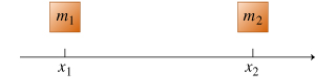# Problem: The blocks shown can only move along the  x-axis.(a) Their velocities at a certain moment are  v1  and  v2 . Find the velocity of the center of mass  vcm  at that moment. Keep in mind that, in general:  vx = dx/dt. Give your answer in terms of  m1,  m2,  v1, and  v2.(b) The blocks' momenta at a certain moment are P1 and P2 . Find the  x  component of the velocity of the center of mass  vcm  at that moment. Give your answer in terms of  m1,  m2, P1, and P2.(c) The blocks' accelerations at a certain moment are  a1  and  a2 . Find the acceleration of the center of mass  acm  at that moment. Keep in mind that, in general,  ax = dvx/dt. Give your answer in terms of  m1,  m2,  a1, and  a2.

###### FREE Expert Solution

(a) Center of mass velocity, vcm = dxcm/dt

xcm = (m1x1 + m2x2)/(m1 + m2)

$\begin{array}{rcl}{\mathbf{v}}_{\mathbf{cm}}& \mathbf{=}& \frac{\mathbf{d}}{\mathbf{dt}}\mathbf{\left(}\frac{{\mathbf{m}}_{\mathbf{1}}{\mathbf{x}}_{\mathbf{1}}\mathbf{+}{\mathbf{m}}_{\mathbf{2}}{\mathbf{x}}_{\mathbf{2}}}{{\mathbf{m}}_{\mathbf{1}}\mathbf{+}{\mathbf{m}}_{\mathbf{2}}}\mathbf{\right)}\\ & \mathbf{=}& \mathbf{\left(}\frac{\mathbf{1}}{{\mathbf{m}}_{\mathbf{1}}\mathbf{+}{\mathbf{m}}_{\mathbf{2}}}\mathbf{\right)}\frac{\mathbf{d}}{\mathbf{dt}}\mathbf{\left(}{\mathbf{m}}_{\mathbf{1}}{\mathbf{x}}_{\mathbf{1}}\mathbf{+}{\mathbf{m}}_{\mathbf{2}}{\mathbf{x}}_{\mathbf{2}}\mathbf{\right)}\\ & \mathbf{=}& \mathbf{\left(}\frac{\mathbf{1}}{{\mathbf{m}}_{\mathbf{1}}\mathbf{+}{\mathbf{m}}_{\mathbf{2}}}\mathbf{\right)}\mathbf{\left(}{\mathbf{m}}_{\mathbf{1}}{\mathbf{v}}_{\mathbf{1}}\mathbf{+}{\mathbf{m}}_{\mathbf{2}}{\mathbf{v}}_{\mathbf{2}}\mathbf{\right)}\end{array}$###### Problem Details

The blocks shown can only move along the  x-axis.
(a) Their velocities at a certain moment are  v1  and  v2 . Find the velocity of the center of mass  vcm  at that moment. Keep in mind that, in general:  vx = dx/dt. Give your answer in terms of  m1,  m2,  v1, and  v2.
(b) The blocks' momenta at a certain moment are P1 and P2 . Find the  x  component of the velocity of the center of mass  vcm  at that moment. Give your answer in terms of  m1,  m2, P1, and P2.
(c) The blocks' accelerations at a certain moment are  a1  and  a2 . Find the acceleration of the center of mass  acm  at that moment. Keep in mind that, in general,  a= dvx/dt. Give your answer in terms of  m1,  m2,  a1, and  a2.